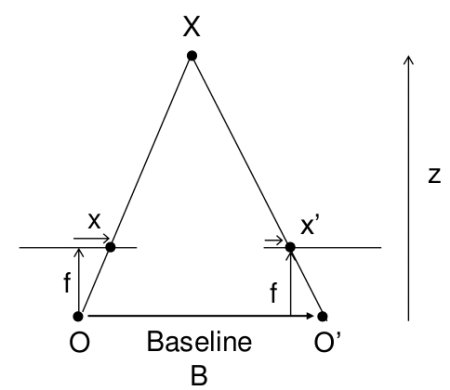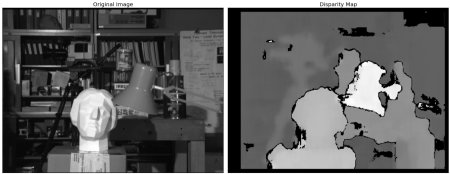### 基础¶disparity = x - x' = \frac{Bf}{Z}

$x$$x'$是图像平面中与场景点3D相对应的点与其相机中心之间的距离。$B$是两个摄像机之间的距离（我们知道），$f$是摄像机的焦距（已经知道）。简而言之，上述方程式表示场景中某个点的深度与相应图像点及其相机中心的距离差成反比。因此，利用此信息，我们可以得出图像中所有像素的深度。

### 代码¶

import numpy as np
import cv2 as cv
from matplotlib import pyplot as plt
stereo = cv.StereoBM_create(numDisparities=16, blockSize=15)
disparity = stereo.compute(imgL,imgR)
plt.imshow(disparity,'gray')
plt.show()### 附加资源¶

• Ros stereo img processing wiki page

### 练习¶

1. OpenCV样本包含生成视差图及其3D重建的示例。查看OpenCV-Python示例代码stereo_match.py​​